# List of unsolved problems in mathematics – Trinh Do

“At first, you should read this paragraph. I want to let you know what mathematics is. After that, I will show you a list of unsolved problems in mathematics. Are these too hard for you so as to solve? The answer depends on your thoughts. If you really love math and have spent a lot of time on doing math research. I think you can solve them someday, but not really everyone, even me. Addtionally, your achievement still depends on your luckiness”Mathematics is the abstract study of topics such as quantity (numbers), structure, space, and change. There is a range of views among mathematicians and philosophers as to the exact scope and definition of mathematics.

Mathematicians seek out patterns and use them to formulate new conjectures. Mathematicians resolve the truth or falsity of conjectures by mathematical proof. When mathematical structures are good models of real phenomena, then mathematical reasoning can provide insight or predictions about nature. Through the use of abstraction and logic, mathematics developed from counting, calculation, measurement, and the systematic study of the shapes and motions of physical objects. Practical mathematics has been a human activity for as far back as written records exist. The research required to solve mathematical problems can take years or even centuries of sustained inquiry.Rigorous arguments first appeared in Greek mathematics, most notably in Euclid’s Elements. Since the pioneering work ofGiuseppe Peano (1858–1932), David Hilbert (1862–1943), and others on axiomatic systems in the late 19th century, it has become customary to view mathematical research as establishing truth by rigorous deduction from appropriately chosenaxioms and definitions. Mathematics developed at a relatively slow pace until the Renaissance, when mathematical innovations interacting with new scientific discoveries led to a rapid increase in the rate of mathematical discovery that has continued to the present day.

Galileo Galilei (1564–1642) said, “The universe cannot be read until we have learned the language and become familiar with the characters in which it is written. It is written in mathematical language, and the letters are triangles, circles and other geometrical figures, without which means it is humanly impossible to comprehend a single word. Without these, one is wandering about in a dark labyrinth.” Carl Friedrich Gauss (1777–1855) referred to mathematics as “the Queen of the Sciences”.Benjamin Peirce (1809–1880) called mathematics “the science that draws necessary conclusions”. David Hilbert said of mathematics: “We are not speaking here of arbitrariness in any sense. Mathematics is not like a game whose tasks are determined by arbitrarily stipulated rules. Rather, it is a conceptual system possessing internal necessity that can only be so and by no means otherwise.” Albert Einstein (1879–1955) stated that “as far as the laws of mathematics refer to reality, they are not certain; and as far as they are certain, they do not refer to reality.” French mathematician Claire Voisin states “There is creative drive in mathematics, it’s all about movement trying to express itself.”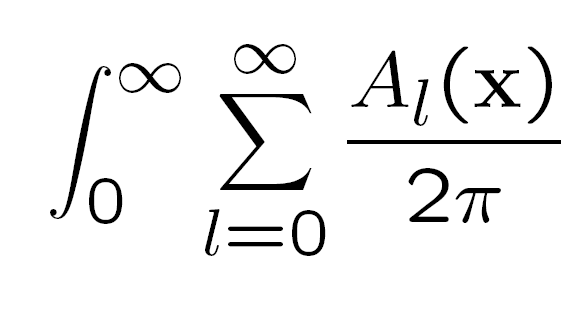Mathematics is used throughout the world as an essential tool in many fields, including natural science, engineering, medicine, finance and the social sciences. Applied mathematics, the branch of mathematics concerned with application of mathematical knowledge to other fields, inspires and makes use of new mathematical discoveries, which has led to the development of entirely new mathematical disciplines, such as statistics and game theory. Mathematicians also engage in pure mathematics, or mathematics for its own sake, without having any application in mind. There is no clear line separating pure and applied mathematics, and practical applications for what began as pure mathematics are often discovered.

This article lists some unsolved problems in mathematics. See individual articles for details and sources.

## Millennium Prize Problems

Of the seven Millennium Prize Problems set by the Clay Mathematics Institute, six have yet to be solved:

The seventh problem, the Poincaré conjecture, has been solved. The smooth four-dimensional Poincaré conjecture is still unsolved. That is, can a four-dimensional topological sphere have two or more inequivalent smooth structures?## Other still-unsolved problems

### Combinatorics

• Number of magic squares (sequence A006052 in OEIS)
• Finding a formula for the probability that two elements chosen at random generate the symmetric group• Frankl’s union-closed sets conjecture: for any family of sets closed under sums there exists an element (of the underlying space) belonging to half or more of the sets
• The Lonely runner conjecture: if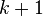runners with pairwise distinct speeds run round a track of unit length, will every runner be “lonely” (that is, be at least a distancefrom each other runner) at some time?
• Singmaster’s conjecture: is there a finite upper bound on the multiplicities of the entries greater than 1 in Pascal’s triangle?
• The 1/3–2/3 conjecture: does every finite partially ordered set contain two elements x and y such that the probability that x appears before y in a random linear extension is between 1/3 and 2/3?
• Conway’s thrackle conjecture

### Discrete geometry

• Solving the Happy Ending problem for arbitrary• Finding matching upper and lower bounds for K-sets and halving lines
• The Hadwiger conjecture on covering n-dimensional convex bodies with at most 2n smaller copies

### Dynamical system

• Furstenberg conjecture – Is every invariant and ergodic measure for the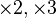action on the circle either Lebesgue or atomic?
• Margulis conjecture — Measure classification for diagonalizable actions in higher-rank groups

### Model theory

• Vaught’s conjecture
• The Cherlin-Zilber conjecture: A simple group whose first-order theory is stable in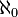is a simple algebraic group over an algebraically closed field.
• The Main Gap conjecture, e.g. for uncountable first order theories, for AECs, and for-saturated models of a countable theory.
• Determine the structure of Keisler’s order
• The stable field conjecture: every infinite field with a stable first-order theory is separably closed.
• Is the theory of the field of Laurent series overdecidable? of the field of polynomials over?
• (BMTO) Is the Borel monadic theory of the real order decidable? (MTWO) Is the monadic theory of well-ordering consistently decidable?
• The Stable Forking Conjecture for simple theories
• For which number fields does Hilbert’s tenth problem hold?
• Assume K is the class of models of a countable first order theory omitting countably many types. If K has a model of cardinalitydoes it have a model of cardinality continuum?
• Is there a logic satisfying the interpolation theorem which is compact?
• If the class of atomic models of a complete first order theory is categorical in the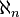, is it categorical in every cardinal?
• Is every infinite, minimal field of characteristic zero algebraically closed? (minimal = no proper elementary substructure)
• Kueker’s conjecture
• Does there exist an o-minimal first order theory with a trans-exponential (rapid growth) function?
• Lachlan’s decision problem
• Does a finitely presented homogeneous structure for a finite relational language have finitely many reducts?
• Do the Henson graphs have the finite model property? (e.g. triangle-free graphs)
• The universality problem for C-free graphs: For which finite sets C of graphs does the class of C-free countable graphs have a universal member under strong embeddings?
• The universality spectrum problem: Is there a first-order theory whose universality spectrum is minimum?

### Ramsey theory

• The values of the Ramsey numbers, particularly• The values of the Van der Waerden numbers

## See also

Source: Wikipedia